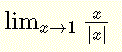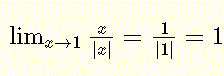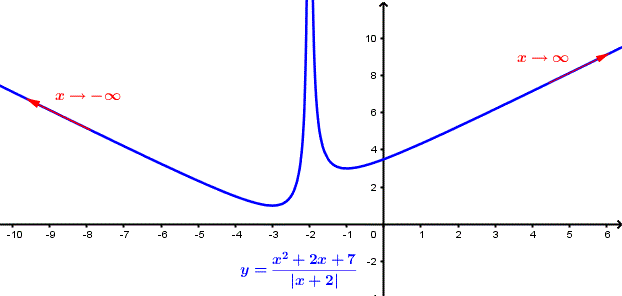# Limits of Absolute Value Functions Questions

How to find the limits of absolute value functions; several examples and detailed solutions are presented along with graphical interpretations. A set of exercises with answers is presented at the bottom of the page.

## Find the limits of the following functions.

### Question

Find the limit:Solution
The steps to find the limit are:### Question

Evaluate the limit:Solution
The steps to find the limit are### Question

Find the limit: $$\lim_{x \to 0} \frac{x}{|x|}$$
Solution
$$\lim_{x \to 0} \frac{x}{|x|} = \frac{0}{|0|} \; \; \text{indeterminate form}$$
Recall that
$$| x | = x$$ for $$x \le 0$$ and $$| x | = - x$$ for $$x \lt 0$$
Let us calculate the limit from the left of $$x = 0$$ where $$x \lt 0$$ and therefore $$| x | = - x$$
$$\lim_{x \to 0^-} \frac{x}{|x|} = \lim_{x \to 0^-} \frac{x}{- x} = \lim_{x \to 0^-} - 1 = -1$$
Let us calculate the limit from the right of $$x = 0$$ where $$x \gt 0$$ and therefore $$| x | = x$$
$$\lim_{x \to 0^+} \frac{x}{|x|} = \lim_{x \to 0^+} \frac{x}{x} = \lim_{x \to 0^+} 1 =1$$
The limits from the left and from the right of x = 0 are not equal, therefore
$$\lim_{x \to 0} \frac{x}{|x|} \; \; \text{does not exist}$$
The graph of f(x) = x / |x| is shown below and we clearly see that the limits from the left and right of 0 are not equal.

### Question

Evaluate the limit: $$\lim_{x \to \infty} \frac{x}{|x|}$$
Solution
as x increases indefinitely , x > 0 and therefore |x| = x
Hence
$$\lim_{x \to \infty} \frac{x}{|x|} = \lim_{x \to \infty} \frac{x}{x} = \lim_{x \to \infty} 1 = 1$$

### Question

Find the limit: $$\lim_{x \to -\infty} \frac{x}{|x|}$$
Solution
as x decreases indefinitely , x < 0 and therefore |x| = - x
Hence
$$\lim_{x \to -\infty} \frac{x}{|x|} = \lim_{x \to -\infty} \frac{x}{ - x} = \lim_{x \to \infty} - 1 = - 1$$

### Question

Does the limit $$\lim_{x \to - 2} \frac{|x + 2|}{x + 2}$$ exists?
Solution
$$\lim_{x \to - 2} \frac{|x + 2|}{x + 2} = \lim_{x \to - 2} \frac{|- 2 + 2|}{- 2 + 2} = \dfrac{0}{0} \;\; \text{indeterminate}$$
Let us calculate the limit from the left of -2 where x ≤ - 2 and from the right of -2 where x ≥ - 2 separateley.
Recall that
If x + 2 ≥ 0 or x ≥ - 2 then | x + 2 | = x + 2
and
If x + 2 ≤ 0 or x ≤ - 2 then | x + 2 | = - $$( x + 2 )$$
Let us calculate the limit from the left of x = - 2.
$$\lim_{x \to - 2^-} \frac{|x + 2|}{x + 2} = \lim_{x \to -2^-} \frac{-(x + 2)}{x + 2} = \lim_{x \to -2^-} - 1 = -1$$
Let us calculate the limit from the right of x = - 2
$$\lim_{x \to - 2^+} \frac{|x + 2|}{x + 2} = \lim_{x \to - 2^+} \frac{x + 2}{x + 2} = \lim_{x \to -2^+} 1 = 1$$
The limits from the left and from the right of x = - 2 are not equal, therefore
$$\lim_{x \to - 2} \frac{|x + 2|}{x + 2} \; \; \text{does not exist}$$

### Question

Find the limit: $$\lim_{x \to 1} \frac{x^2+2x-3}{|x - 1|}$$
Solution
$$\lim_{x \to 1} \frac{x^2+2x-3}{|x - 1|} = \frac{(1)^2+2(1)-3}{|(1) - 1|} = \dfrac{0}{0} \; \; \text{indeterminate}$$
At x = 1 both numerator and denominator are equal to zero, they therefore have a common factor x - 1. We factor the numerator.
$$\lim_{x \to 1} \frac{x^2+2x-3}{|x - 1|} = \lim_{x \to 1} \frac{(x-1)(x+3)}{|x - 1|}$$
Recall that
| x - 1 | = x - 1 for x - 1 ≥ 0 or x ≥ 1
and
| x - 1 | = - $$( x - 1 )$$ for x - 1 < 0 or x < 1
Let us calculate the limit from the left of x = 1
$$\lim_{x \to 1^-} \frac{x^2+2x-3}{|x - 1|} = \lim_{x \to 1^-} \frac{(x-1)(x+3)}{-(x-1)} = \lim_{x \to 1^-} - (x + 3) = - 4$$
Let us calculate the limit from the right of x = 1
$$\lim_{x \to 1^+} \frac{x^2+2x-3}{|x - 1|} = \lim_{x \to 1^+} \frac{(x-1)(x+3)}{x-1} = \lim_{x \to 1^-} (x + 3) = 4$$
The limits from the left and from the right are not equal, therefore
$$\lim_{x \to 1} \frac{x^2+2x-3}{|x - 1|} \; \; \text{does not exist}$$
The graph of f(x) = (x^2 + 2 x - 3)/|x - 1| is shown below and we clearly see that the limits from the left and right of 1 are not equal.Figure 2. Graph of f(x) = (x2 + 2 x - 3)/|x - 1|.

### Question

Evaluate the limit: $$\lim_{x \to \infty} \frac{x^2+5x+7}{|x + 2|}$$
Solution
As x increases indefinitely, x + 2 also increases indefinitely and therefore x + 2 > 0, hence
$$\lim_{x \to \infty} \frac{x^2+5x+7}{|x + 2|} = \lim_{x \to \infty} \frac{x^2+5x+7}{x + 2} = + \infty$$

### Question

Find the limit: $$\lim_{x \to - \infty} \frac{x^2+5x+7}{|x + 2|}$$
Solution
As x decreases indefinitely, x + 2 also decreases indefinitely and therefore x + 2 < 0, hence
$$\lim_{x \to -\infty} \frac{x^2+5x+7}{|x + 2|} = \lim_{x \to -\infty} \frac{x^2+5x+7}{-(x + 2)} = + \infty$$
The graph of $$f(x) = (x^2 + 5 x + 7)/|x + 2|$$ is shown below and we clearly see that $$y = f(x)$$ increases indefinitely as x increases indefinitely and also as x decreases indefinitely.Figure 3. Graph of $$f(x) = \dfrac {x^2 + 5 x + 7}{|x + 2|}$$.

## Exercises

Find the limits
1) $$\lim_{x \to 0} \frac{x^2}{|x|}$$
2) $$\lim_{x \to - 6^-} \frac{-(x + 6)}{|x + 6|}$$
3) $$\lim_{x \to - 6^+} \frac{-(x + 6)}{|x + 6|}$$
4) $$\lim_{x \to 3} \frac{x^2-x-6}{|x - 3|}$$

1) 0
2) 1
3) - 1
4) does not exist